# Samacheer Kalvi Books: Tamilnadu State Board Text Books Solutions

## Samacheer Kalvi 11th Computer Science Chapter 6 Specification and Abstraction Notes PDF Download: Tamil Nadu STD 11th Computer Science Chapter 6 Specification and Abstraction NotesSamacheer Kalvi 11th Computer Science Chapter 6 Specification and Abstraction Notes PDF Download: Tamil Nadu STD 11th Computer Science Chapter 6 Specification and Abstraction Notes

## Samacheer Kalvi 11th Computer Science Chapter 6 Specification and Abstraction Notes PDF Download

We bring to you specially curated Samacheer Kalvi 11th Computer Science Chapter 6 Specification and Abstraction Notes PDF which have been prepared by our subject experts after carefully following the trend of the exam in the last few years. The notes will not only serve for revision purposes, but also will have several cuts and easy methods to go about a difficult problem.

 Board Tamilnadu Board Study Material Notes Class Samacheer Kalvi 11th Computer Science Subject 11th Computer Science Chapter Chapter 6 Specification and Abstraction Format PDF Provider Samacheer Kalvi Books

## How to Download Samacheer Kalvi 11th Computer Science Chapter 6 Specification and Abstraction Notes PDFs?

2. Click on the Samacheer Kalvi 11th Computer Science Notes PDF.
3. Look for your preferred subject.
4. Now download the Samacheer Kalvi 11th Computer Science Chapter 6 Specification and Abstraction notes PDF.

## Download Samacheer Kalvi 11th Computer Science Chapter 6 Specification and Abstraction Chapterwise Notes PDF

PART – 1

11th Computer Science Chapter 6 Book Back Answers Question 1.
Which of the following activities is algorithmic in nature?
(a) Assemble a bicycle
(b) Describe a bicycle
(c) Label the parts of a bicycle
(d) Explain how a bicycle works
(a) Assemble a bicycle

Question 2.
Which of the following activities is not algorithmic in nature?
(a) Multiply two numbers
(b) Draw a kolam
(c) Walk in the park
(d) Braid the hair
(d) Braid the hair

Question 3.
Omitting details inessential to the task and representing only the essential features of the task is known as ………………..
(a) specification
(b) abstraction
(c) composition
(d) decomposition
(b) abstraction

Question 4.
Stating the input property and the input-output relation a problem is known ………………..
(a) specification
(b) statement
(c) algorithm
(d) definition
(a) specification

Question 5.
Ensuring the input-output relation is ………………..
(a) the responsibility of an algorithm and the right of the user.
(b) the responsibility of the user and the right of the algorithm.
(c) the responsibility of the algorithm but not the right of the user.
(d) the responsibility of both the user and the algorithm.
(a) the responsibility of an algorithm and the right of the user.

Question 6.
If i = 5 before the assignment i : = i – 1 after the assignment, the value of i is ………………..
(a) 5
(b) 4
(c) 3
(d) 2
(b) 4

Question 7.
If O < i before the assignment i : = i – 1 after the assignment, we can conclude that
(a) 0 < i
(b) 0 = i
(c) i = 0
(d) 0 = i
(b) 0 = i

PART – 2

Question 1.
Define an algorithm.
An algorithm is a sequence of instructions to accomplish a task or solve a problem.

Question 2.
Distinguish between an algorithm and a process.
Algorithm:

• An Algorithm is a sequence of instructions to solve a problem.
• Elements of a process are abstracted in algorithms.
• Example: An algorithm can be compared to a recipe

Process:

• A process is the execution of instructions to accomplish the intended task.
• A process is the actual execution of the steps to a solution.
• Example: A process can be compared to cooking.

Question 3.
Initially, farmer, goat, grass, wolf = L, L, L, L and the farmer crosses the river with goat. Model the action with an assignment statement. Model of the action with an assignment statement:

1. — farmer, goat,grass,wolf = L,L,L,L.
2. Farmer,goat: = R,R
3. – farmer,goat,grass,wolf = R,R,L,L
4. – farmer: = L
5. — farmer,goat,grass,wolf = L,R,L,L
6. farmer,grass: = R,R
7. — farmer,goat,grass,wolf = R,R,R,L
8. farmer,goat: = L,L
9. — farmer,goat,grass, wolf = L,L,R,L
10. farmer, wolf: = R,R
11. — farmer,goat,grass, wolf = R,L,R,R
12. farmer: = L
13. — farmer,goat,grass,wolf = L,L,R,R
14. farmer, goat: = R,R
15. — farmer,goat,grass,wolf = R,R,R,R

Question 4.
Specify a function to find the minimum of two numbers.
Function specification:
–minimum (a,b)
–inputs: a and b
–outputs: a is minimum such that a < b
b is minimum such that b<a

Question 5.
If 2–√ = 1.414, and the square_root() function returns – 1.414, does it violate the following specification?

1. – – square – root(x)
2. – – inputs: x is a real number, x > 0
3. – – outputs: y is a real number such that y2 = x

No, because (- 1.414)2 = 2, which satisfies input – output relation (y2 = x)

PART – 3

Question 1.
When do you say that a problem is algorithmic in nature?
We usually say that a problem is algorithmic in nature when its solution involves the construction of an algorithm. Some types of problems can be immediately recognized as algorithmic.
Example: The well-known goat, grass, and wolf problem.

Question 2.
What is the format of the specification of an algorithm?
Let P be the required property of the inputs and Q the property of the desired outputs.
Format of specification of an algorithm:

1. Algorithm name (Inputs)
2. Inputs – P
3. Outputs – Q

Question 3.
What is abstraction?

Abstraction:
A problem can involve a lot of details. Several of these details are unnecessary for solving the problem. Only a few details are essential.

Ignoring or hiding unnecessary details and modeling an entity only by its essential properties is known as abstraction.

For example, when we represent the state of a process, we select only the variables essential to the problem and ignore inessential details.

Question 4.
How is the state represented in algorithms?
The state represented in an algorithm is a set of variables. At any place, the value of the variables are substituted and evaluation is done.

Question 5.
What is the form and meaning of the assignment statement?
The assignment statement is used to assign values to the variables.
The variable on the left side of the assignment operator and a value on the right side.
Variable : = Value

Question 6.
What is the difference between assignment operator and equality operator?
Assignment Operator:
The assignment operator is used to assign the values to the variable.

Equality Operator:
The equality operator is used to compare the values of both the variables and the result is true or false.

PART – 4
IV. Explain in Detail

Question 1.
Write the specification of an algorithm hypotenuse whose inputs are the lengths of the two shorter sides of a right-angled triangle, and the output is the length of the third side.

1. hypotenuse (a, b)
2. – – inputs: a, b are real numbers, a > 0, b > 0
3. – – outputs: c2 = a2 + b2 where c is real number, c > 0

Question 2.
Suppose you want to solve the quadratic equation ax2 + bx + c – 0 by an algorithm. quadratic_solve (a, b, c)
— inputs:?
— outputs:?
You intend to use the formula and you are prepared to handle only real number roots. Write a suitable specification.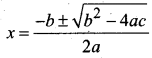1. Quadratic – solve (a, b, c)
2. – – inputs: b2 – 4 ac > = 0 where a, b, c are real numbers, a ? 0
3. – – outputs: and x2 are real numbers such that a(x1)2 + bx1 + c = 0 and a(x2)2 + bx2 + c = 0

Question 3.
Exchange the contents: Given two glasses marked A and B. Glass A is full of apple drink and glass B is full of grape drink. For exchanging the contents of glasses A and B, represent the state by suitable variables, and write the specification of the algorithm.
Let the variables a, b, c represent glass A, glass B, and Glass C respectively. Variables A, B, C can store values APPLE, GRAPE, or EMPTY.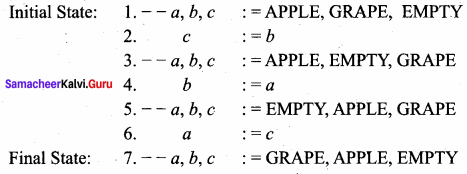Specification:

1. Exchange ( )
2. – – inputs: a, b, c := APPLE, GRAPE, EMPTY
3. – – outputs: a, b,c: = GRAPE, APPLE, EMPTY

### Samacheer Kalvi 11th Computer Science Specification and Abstraction Additional Questions and Answers

PART – 1

Question 1.
A(n) _________ is a sequence of instructions to accomplish a task or solve a problem.
a) pseudo-code
b) flow chart
c) algorithm
d) None of these
c) algorithm

Question 2.
Who was a Hungarian Mathematician?
(a) G. Polya
(b) John Wiley
(c) Krysia Broda
(d) Steve Vickers
(a) G. Polya

Question 3.
A computer can only execute instructions in a _________
a) pseudo-code
b) procedure
c) block
d) programming language
d) programming language

Question 4.
……………….. state the properties of the given input and the relation between the input and the output.
(a) Composition
(b) Abstraction
(c) Decomposition
(d) Specification
(d) Specification

Question 5.
Identify the correct statement from the following.
a) A problem is specified by given input data, desired output data, and a relation between the input and the output data
b) G Polya made fundamental contributions to number theory, numerical analysis, and probability theory
c) An algorithm is a sequence of statements.
d) All the above
d) All the above

Question 6.
What is the specification of the algorithm?
(a) divide (A + B)
(b) square – root (n)
(c) square – root (x)
(b) square – root (n)

Question 7.
We can construct algorithms using basic building block as _________
a) Data and Variables
b) Control flow
c) Functions
d) All the above
d) All the above

Question 8.
The data stored in a variable is also called a ………………..
(a) process
(b) data
(c) value
(d) constant
(c) value

Question 9.
__________is a named box for storing data,
a) Function
b) Algorithm
c) Constant
d) Variable
d) Variable

Question 10.
……………….. is the left side of the assignment.
(a) variable
(b) value
(c) operator
(d) all the above
(c) operator

Question 11.
We can store a value in a variable or change the value of variable, using a(n) __________statement.
a) assignment
b) output
c) control
d) None of these
a) assignment

Question 12.
If i: = 2 before the assignment, then i: = i * 3 after the assignment is ………………..
(a) 6
(b) 5
(c) 0
(d) 1
(a) 6

Question 13.
The order of execution of statements in an algorithm is known as the
a) control flow
b) iteration
c) fetching
d) None of these
a) control flow

Question 14.
If – – m, n = 22, 5 and m, n : = m + 3, n – 1 then the value of m and n are ………………..
(a) 25, 4
(b) 24,5
(c) 22, 5
(d) 23,21
(a) 25, 4

Question 15.
In ___________ control flow, a sequence of statements is executed one after another in the same order as they are written.
a) alternative
b) iterative
c) random
d) sequential
d) sequential

Question 16.
What are the values of variables m and n after the assignments in line (1) and line (3)?
1. m, n : = 2, 5
2. – – m, n = ?, ?
3. m, n : = m + 3, n – 1
4. – – m, n = ?, ?
(a) 4, 5
(b) 5, 4
(c) 5,5
(d) 4, 4
(b) 5, 4

Question 17.
In ___________ control flow, a condition of the state is tested, and if the condition is true, a statement is executed.
a) alternative
b) iterative
c) random
d) sequential
b) iterative

Question 18.
which one of the following is the assignment operator?
(a) =
(b) = =
(c) + = =
(d) + +
(a) =

Question 19.
There are ___________ basic principles and techniques for designing algorithms.
a) 5
b) 6
c) 4
d) 3
c) 4

Question 20.
which one of the following is the comment line in algorithm?
(a) + +
(b) 1 1
(c) – –
(d) = =
(c) – –

Question 21.
A problem is specified in terms of the __________
a) input given
b) output desired
c) Both A and B
d) None of the above
c) Both A and B

Question 22.
The values of the variables when the algorithm starts is ………………..
(a) stage
(b) initial stage
(c) initial state
(d) starting state
(c) initial state

Question 23.
Identify the correct statement from the following.
a) The specification must state the properties of the given input, and the relation between the input and the output
b) When we represent the state of a process, we select only the variables essential to the problem and ignore inessential details
c) A control flow statement tests a condition of the state and, depending on the value of the condition, decides the next statement be executed
d) All the above
d) All the above

Question 24.
Instructions of a computer are also known as ………………..
(a) commands
(b) statements
(c) Abstractions
(d) Functions
(b) statements

Question 25.
Divide the main algorithm into functions are called__________
a) Specification
b) Abstraction
c) Decomposition
d) All the above
c) Decomposition

Question 26.
Which are the named boxes used for storing data?
(a) data
(b) variables
(c) control flow
(d) functions
(b) variables

Question 27.
Identify the incorrect statement from the following.
a) A problem is specified by the given input and the desired output
b) When we use the functions, it is enough to know the specification of the function
c) It is necessary to know how the function is implemented
d) The goal of the algorithm is to establish the relation between the input and the desired output
c) It is necessary to know how the function is implemented

Question 28.
The order of execution of statement is called
(a) Composition
(b) Functions
(c) Control flow
(d) Specifications
(c) Control flow

Question 29.
The inputs and outputs are passed between an algorithm and the user through__________
a) constants
b) variables
c) operators
d) expressions
b) variables

Question 30.
In which one of the following control flows, the statements are repeated until the condition becomes false ………………..
(a) Sequential
(b) iterative
(c) selection
(d) alternative
(b) iterative

Question 31.
The values of the variables when the algorithm finishes is known as the__________ state.
a) initial
b) final
c) intermediate
d) None of these
b) final

Question 32.
A is like a sub-algorithm.
(a) function
(b) data
(c) variable
(d) state
(a) function

Question 33.
__________is the specification format.
a) The name of the algorithm and the inputs
b) Input: the property of the inputs
c) Output: the desired input-output relation
d) All the above
d) All the above

Question 34.
Fill in the box given below figure ………………..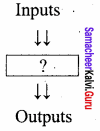(a) processing
(b) solving
(c) algorithm
(d) functions
(c) algorithm

Question 35.
__________is the most effective mental tool used for managing complexity.
a) Abstraction
b) Composition
c) Decomposition
d) All the above
a) Abstraction

Question 36.
The first part of the specification is ………………..
(a) Input
(b) Output
(c) property of inputs
(d) The name of the algorithm and the inputs
(d) The name of the algorithm and the inputs

Question 37.
When an algorithm is very complex, we can decompose it into __________
a) expressions
b) variables
c) functions
d) None of these
c) functions

Question 38.
In multiple variable assignment statement, the number of variables and the number of expressions must ………………..
(a) differ at least by 1
(b) not equal to 1
(c) equal to 0
(d) be equal
(d) be equal

Question 39.
Identify the correct statement from the following.
a) Specification abstracts a problem by the essential variables of the problem.
b) The values of the variables in an algorithm define the state of the process.
c) Assignment statement changes the values of variables, and hence the state.
d) All the above
b) The values of the variables in an algorithm define the state of the process.

Question 40.
To execute in a computer, an algorithm must be expressed using the statement of ………………..
(a) Object code
(b) Machine Language
(c) Assembler
(d) Programming Languages
(d) Programming Languages

Question 41.
If the variable already has a value, and you are assigning a new value, what will happen to the old variable?
(a) retained
(c) lost
(d) becomes 0
(c) lost

Question 42.
Identify the function name: square (A + B) ………………..
(a) A
(b) B
(c) A + B
(d) square
(d) square

Question 43.
Identify the correct statement ………………..
(a) The first part of the specification is the name of the algorithm with input
(b) the Second one is input properties with comments
(c) Third one is the relation between input-output
(d) All the three are correct statements
(d) All the three are correct statements

Question 44.
Find the wrong statement from the following ………………..
(a) The state of a process can be represented by a set of variables in an algorithm
(b) The state at any point of execution is simply the values of the variables at that point.
(c) As the values of the variables are changed, the state remains constant.
(d) As a process evolves, the state changes.
(c) As the values of the variables are changed, the state remains constant.

Question 45.
The input and output are passed between an algorithm and the user through ………………..
(a) data
(b) assignment
(c) stage
(d) variables
(d) variables

Question 46.
Match the following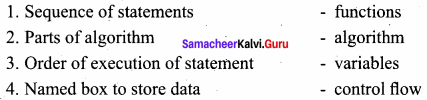(a) 2, 1, 4, 3
(b) 1, 2, 3, 4
(c) 4,3,2, 1
(d) 4, 2, 3, 1
(a) 2, 1, 4, 3

Question 47.
Match the following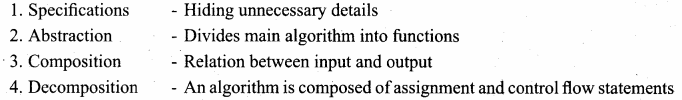(a) 3, 1, 4, 2
(b) 1,2, 3, 4
(c) 4,3,2, 1
(d) 4, 2, 3, 1
(a) 3, 1, 4, 2

Question 48.
Which one of the following statements is used to annotate a program for human readers?
(b) state
(c) variables
(d) functions

Question 49.
In the specification, the input and output can be written using ………………..
(a) English
(b) Mathematical notation
(c) Binary format
(d) Both a and b
(d) Both a and b

Question 50.
Which one of the following defines the rights and responsibilities of the designer and the user?
(a) Abstraction
(b) Composition
(c) Decomposition
(d) Specification
(d) Specification

Question 51.
Which one of the following is the most effective mental tool used for managing complexity?
(a) Specification
(b) Abstraction
(c) Composition
(d) Decomposition
(b) Abstraction

Question 52.
How will be the input and output passed between an algorithm and the user ………………..
(b) Assignment
(c) Variable
(d) Functions
(c) Variable

Question 53.
The goal of the algorithm is ………………..
(a) to state the input properties
(b) to state the output properties
(c) to divide into functions
(d) to establish the input-output relation
(d) to establish the input-output relation

PART – 2

Question 1.
Define Algorithm.
An algorithm is a sequence of instructions to accomplish a task or solve a problem.

Question 2.
Mention the three different types of Chameleons.
There are three different types of chameleons: red chameleons, green chameleons, and blue chameleons.

Question 3.
What are the steps to be followed when solving a mathematical problem?

• Understand the problem.
• Devise a plan.
• Carry out the plan.

Question 4.
Write the basic building blocks of algorithms.

1. Data
2. Variables
3. Control flow
4. Functions

Question 5.
What is data? Explain the suitable example.
Data:
Algorithms take input data, process the data, and produce output data. Computers provide instructions to perform operations on data.
For example, there are instructions for doing arithmetic operations on numbers, such as add, subtract, multiply and divide. There are different kinds of data such as numbers and text.

Question 6.
What are the basic principles and techniques for designing Algorithms?

1. Specification
2. Abstraction
3. Composition
4. Decomposition

PART – 3
III. Explain in Brief

Question 1.
What do you mean by the algorithmic problem?
There are some principles and techniques for constructing algorithms. We usually say that a problem is algorithmic in nature when its solution involves the construction of an algorithm. Some types of problems can be immediately recognized as algorithmic.

Question 2.
What is Decomposition?
We divide the main algorithm into functions. We construct each function independently of the main algorithm and other functions. Finally, we construct the main algorithm using the functions. When we use the functions, it is enough to know the specification of the function. It is not necessary to know how the function is implemented.

Question 3.
Justify the Chameleons of Chromeland problem is algorithmic.
The Chameleons of Chromeland problem:
On the island of Chromeland, there are three different types of chameleons: red chameleons, green chameleons, and blue chameleons. Whenever two chameleons of different colors meet, they both change color to the third color. For which a number of red, green, and blue chameleons is it, possible to arrange a series of meetings that results in all the chameleons displaying the same color? This is an algorithmic problem because there is an algorithm to arrange meetings between chameleons.

Question 4.
Define functions.
The parts of an algorithm are known as functions. A function is like a sub-algorithm. It takes, an input, and produces an output, satisfying a desired input-output relation.

Question 5.
Explain the state with an example.
Computational processes in the real-world have stated. As a process evolves, the state changes.
How do we represent the state of a process and the change of state, in an algorithm? The state of a process can be represented by a set of variables in an algorithm.
The state at any point of execution is simply the values of the variables at that point. As the values of the variables are changed, the state changes.

Question 6.
What is the desired relation between the inputs A and B, and the output q and r?
1. The two outputs q (quotient) and r (remainder) should satisfy the property
A = q X B + r,
and
2. The remainder r should be less than the divisor B,
0 < r < B
Combining these requirements, we will specify the desired input-output relation as
– outputs: A = q X B + r and 0 < r < B.

PART – 4
IV. Explain in Detail

Question 1.
Explain control with its types.
An algorithm is a sequence of statements. However, after executing a statement, the next statement executed need not be the next statement in the algorithm. The statement to be executed next may depend on the state of the process. Thus, the order in which the statements are executed may differ from the order in which they are written in the algorithm. This order of execution of statements is known as the control flow.
There are three important control flow statements to alter the control flow depending on the state.

• In sequential control flow, a sequence of statements is executed one after another in the same order as they are written.
• In alternative control flow, a condition of the state is tested, and if the condition is true, one statement is executed; if the condition is false, an alternative statement is executed.
• In iterative control flow, a condition of the state is tested, and if the condition is true, a statement is executed. The two steps of testing the condition and executing the statement are repeated until the condition becomes false.

Question 2.
Explain Algorithm Design Techniques.
There are a few basic principles and techniques for designing algorithms.
1. Specification:
The first step in problem-solving is to state the problem precisely. A problem is specified in terms of the input given and the output desired. The specification must also state the properties of the given input, and the relation between the input and the output.

2. Abstraction:
A problem can involve a lot of details. Several of these details are unnecessary for solving the problem. Only a few details are essential. Ignoring or hiding unnecessary details and modeling an entity only by its essential properties is known as abstraction. For example, when we represent the state of a process, we select only the variables essential to the problem and ignore inessential details.

3. Composition:
An algorithm is composed of assignment and control flow statements. A control flow statement tests a condition of the state and depending on the value of the condition, decides the next statement be executed.

4. Decomposition:
We divide the main algorithm into functions. We construct each function independently of the main algorithm and other functions. Finally, we construct the main algorithm using the functions. When we use the functions, it is enough to know the specification of the function. It is not necessary to know how the function is implemented.

Question 3.
Give an example for the specification. Explain in detail.
The specification of an algorithm to compute the quotient and remainder after dividing an integer A by another integer B is given as follows,

divide (22, 5) = 4, 2
divide (15, 3) = 5,0

Let A and B be the input variables. We will store the quotient in a variable q and the remainder in a variable r. So q and r are the output variables.

What are the properties of inputs A and B?

• A should be an integer. Remainder Is meaningful only for integer division, and
• B should not be 0, since division by 0 is not allowed.

We will specify the properties of the inputs as — inputs: A is an integer and B + 0

What is the desired relation between inputs A and B, and the outputs q and r?

• The two outputs q (quotient) and r (remainder) should satisfy the property A = q x B + r, and
• The remainder r should be less than the divisor B, 0 < r < B

Combining these requirements, we will specify the desired input-output relation as — outputs: A = q x B + r and 0 < r < B.

The comment that starts with — inputs: actually is the property of the given inputs. The comment that starts with — outputs: is the desired relationship between the inputs and the outputs. The specification of the algorithm is;

• divide (A, B).
• inputs: A is an integer and B ≠ 0.
• outputs : A = q X B + r and 0 ≤ r < B.

Question 4.
What are the values of variables m and n after the assignments in line (1) and line (3)?

1. m, n : = 2, 5
2. – – m, n = ?, ?
3. m,n: = m + 3, n – 1
4. – – m, n = ?, ?

The assignment in line (1) stores 2 in variable m, and 5 in variable n.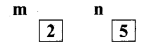The assignment in line (3) evaluates the expressions m + 3 and n – 1 using the current values of m and n as
m + 3 , n – 1
= 2 + 3, 5 – 1
= 5, 4
and stores the values 5 and 4 in the variables m and n, respectively.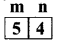1. m, n : = 2, 5
2. – – m, n = 2, 5
3. m, n : = m + 3, n – 1
4. – – m, n = 2 + 3, 5 – 1 = 5, 4

Values of the variables after the two assignments are shown in line (2) and line (4).

Question 5.
Explain the Basic Building Blocks of Algorithms.
Data:
Algorithms take input data, process the data, and produce output data. Computers provide instructions to perform operations on data. For example, there are instructions for doing arithmetic operations on numbers, such as add, subtract, multiply and divide. There are different kinds of data such as numbers and text.

Variables:
Variables are named boxes for storing data. When we do operations on data, We need to store the results in variables. The data stored in a variable is also known as the value of the variable. We can store a value in a variable or change the value of variable, using an assignment statement.

Control flow:
An algorithm is a sequence of statements. However, after executing a statement, the next statement executed need not be the next statement in the algorithm. The statement to be executed next may depend on the state of the process. Thus, the order in which the statements are executed may differ from the order in which they are written in the algorithm. This order of execution of statements is known as the control flow.

There are three important control flow statements to alter the control flow depending on the state.

1. In sequential control flow, a sequence of statements are executed one after another in the same order as they are written.
2. In alternative control flow, a condition of the state is tested, and if the condition is true, one statement is executed; if the condition is false, an alternative statement is executed.
3. In iterative control flow, a condition of the state is tested, and if the condition is true, a statement is executed. The two steps of testing the condition and executing the statement are repeated until the condition becomes false.

Functions:
The parts of an algorithm are known as functions. A function is like a sub-algorithm. It takes an input, and produces an output, satisfying a desired input-output relation.

Question 6.
Explain specification.
Specification:
To solve a problem, first we must state the problem clearly and precisely. A problem is specified by the given input and the desired output. To design an algorithm for solving a problem, we should know the properties of the given input and the properties of the desired output. The goal of the algorithm is to establish the relation between the input and the desired output.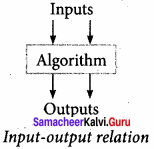An algorithm is specified by the properties of the given input and the relation between the input and the desired output. In simple words, specification of an algorithm is the desired input – output relation. The inputs and outputs are passed between an algorithm and the user through variables. The values of the variables when the algorithm starts is known as the initial state, and the values of the variables when the algorithm finishes is known as the final state.

Let P be the required property of the inputs and Q the property of the desired outputs. Then the algorithm S is specified as

1. algorithm name (inputs)
2. – – inputs : P
3. – -outputs: Q

This specification means that if the algorithm starts with inputs satisfying P, then it will finish with the outputs satisfying Q. A double dash – indicates that the rest of the line is a comment. Comments are statements which are used to annotate a program for human readers and not executed by the computer. Comments at crucial points of flow are useful, and even necessary, to understand the algorithm. In our algorithmic notation, we use double dashes (-) to start a comment line. (In C++, a double slash // indicates that the rest of the line is a comment).

Specification format:
We can write the specification in a standard three-part format:

1. The name of the algorithm and the inputs.
2. Input: the property of the inputs.
3. Output: the desired input-output relation.

The first part is the name of the algorithm and the inputs. The second part is the property of the inputs. It is written as a comment which starts with – inputs: The third part is the desired input-output relation. It is written as a comment which starts with – outputs:. The input and output can be written using English and mathematical notation.

Specification as a contract:
Specification of an algorithm serves as a contract between the designer of the algorithm and the users of the algorithm because it defines the rights and responsibilities of the designer and the user. Ensuring that the inputs satisfy the required properties is the responsibility of the user, but the right of the designer. The desired input-output relation is the responsibility of the designer and the right of the user. Importantly, if the user fails to satisfy the properties of the inputs, the designer is free from his obligation to satisfy the desired input-output relation.

## How to Prepare using Samacheer Kalvi 11th Computer Science Chapter 6 Specification and Abstraction Notes PDF?

Students must prepare for the upcoming exams from Samacheer Kalvi 11th Computer Science Chapter 6 Specification and Abstraction Notes PDF by following certain essential steps which are provided below.

• Use Samacheer Kalvi 11th Computer Science Chapter 6 Specification and Abstraction notes by paying attention to facts and ideas.
• Pay attention to the important topics
• Refer TN Board books as well as the books recommended.
• Correctly follow the notes to reduce the number of questions being answered in the exam incorrectly
• Highlight and explain the concepts in details.

## Frequently Asked Questions on Samacheer Kalvi 11th Computer Science Chapter 6 Specification and Abstraction Notes

#### How to use Samacheer Kalvi 11th Computer Science Chapter 6 Specification and Abstraction Notes for preparation??

Read TN Board thoroughly, make separate notes for points you forget, formulae, reactions, diagrams. Highlight important points in the book itself and make use of the space provided in the margin to jot down other important points on the same topic from different sources.

#### How to make notes for Samacheer Kalvi 11th Computer Science Chapter 6 Specification and Abstraction exam?

Read from hand-made notes prepared after understanding concepts, refrain from replicating from the textbook. Use highlighters for important points. Revise from these notes regularly and formulate your own tricks, shortcuts and mnemonics, mappings etc.
Share: